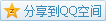# MIT OCW

## 18.02 Multivariable Calculus

### Line Integral

#### Vector Fields

##### Definition

A (2D) vector field is some vector $\mathbf{F}=M\hat\mathbf{i}+N\hat\mathbf{j}$, where $M,N$ depend on $(x,y)$.

##### Demonstration

Usually, we can draw a vector field with some samples (NOT ALL SAMPLES) in a Cartesian plane to demonstrate its direction.

#### Work

Work with a force $\mathbf{F}$ and a shift $\mathbf{s}$ is $W=\mathbf{F}\cdot \mathbf{s}$, and given a position vector $\mathbf{r}$, the distance in a small range becomes $\Delta\mathbf{r}$.
It only holds when $\mathbf{F}$ is constant and $\Delta\mathbf{r}$ has a fixed direction, so if the force $\mathbf{F}$ is a changing vector field and $\mathbf{r}$ is the position vector of some curve $C$, we can use line integral to solve it.
Basically, the idea is to calculate the sum of small work $W=\sum \mathbf{F}\cdot\Delta\mathbf{r}$, where $\Delta\mathbf{r}$ is set to each piece (with direction) of the curve $C$.
If we parameterize the curve $C$ in the form of $x=x(t),y=y(t)$ and by chain rule we can get $$W=\int_{C}\mathbf{F}\frac{\mathrm{d}\mathbf{r}}{\mathrm{d}t}\mathrm{d}t.$$